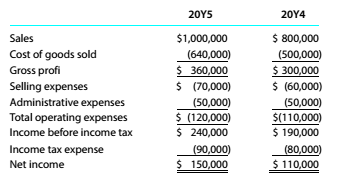Chapter 9, Problem 9.5E

Chapter
Section
Textbook Problem

Horizontal analysis of the income statement Income statement data for Yellowstone Images Inc. for the years ended December 31, 20Y5 and 20Y4, are as follows:a. Prepare a comparative income statement with horizontal analysis, indicating the increase (decrease) for 20Y5 when compared with 20Y4. Round to one decimal place. b. What conclusions can be drawn from the horizontal analysis?

To determine

Concept Introduction:

Horizontal Analysis:

Horizontal Analysis is used to analyze the trend of an item. Horizontal Analysis is done in different periods for example analysis of trend of sales for several years is done using the horizontal analysis.

Requirement-a:

To Prepare:

The Comparative Income Statement using horizontal analysis.

Explanation

The Comparative Income Statement using horizontal analysis is prepared as follows:

 Horizontal Analysis of Comparative Income Statement Increase (Decrease) Year 20Y5 20Y4 Amount Percentage A B C = (A-B) C/B Sales \$ 862,000 \$ 785,000 \$ 77,000 9.8% Cost of Goods Sold \$ 650,000 \$ 500,000 \$ 150,000 30.0% Gross Profit \$ 212,000 \$ 285,000 \$ (73,000) -25.6% Selling expenses \$ 44,000 \$ 40,000 \$ 4,000 10...
To determine

Concept Introduction:

Horizontal Analysis:

Horizontal Analysis is used to analyze the trend of a particular item. Horizontal Analysis is done for items for different periods for example sale trends for several years are found using horizontal analysis.

Requirement-a:

To Indicate:

The results from horizontal analysis.

Still sussing out bartleby?

Check out a sample textbook solution.

See a sample solution

The Solution to Your Study Problems

Bartleby provides explanations to thousands of textbook problems written by our experts, many with advanced degrees!

Get Started

Find more solutions based on key concepts